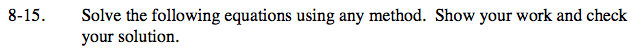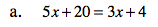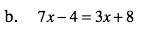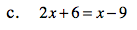Home > CC2 > Chapter Ch8 > Lesson 8.1.1 > Problem8-15

8-15.
1. Solve the following equations using any method. Show your work and check your solution. Homework Help ✎

1. 5x + 20 = 3x + 4

2. 7x − 4 = 3x + 8

3. 2x + 6 = x − 9Once you have found an answer, substitute it for x in the original equation to check if you are correct.

x = −8x = −15